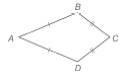Chapter 4.2, Problem 35E### Elementary Geometry for College St...

6th Edition
Daniel C. Alexander + 1 other
ISBN: 9781285195698

#### Solutions

Chapter
Section### Elementary Geometry for College St...

6th Edition
Daniel C. Alexander + 1 other
ISBN: 9781285195698
Textbook Problem
1 views

# For Exercises 32 to 35, consider kite A B C D with A B ¯ ≅ A D ¯ and B C ¯ ≅ D C ¯ .For kite A B C D , A B = x 6 + 5 , A D = x 3 + 3 , and B C = x - 2 . Find the perimeter of A B C D .

To determine

To find:

The perimeter of ABCD.

Explanation

Given,

The kite ABCD with

BC¯DC¯,

AB=x6+5,

BC=x-2

In the given kite, we have

Thus,

x6+5=x3+3

x6+5+-x3=x3+3+-x3

x6+-x3+5=x3+-x3+3

x6+-x3+5=3

x6+-x3

### Still sussing out bartleby?

Check out a sample textbook solution.

See a sample solution

#### The Solution to Your Study Problems

Bartleby provides explanations to thousands of textbook problems written by our experts, many with advanced degrees!

Get Started

#### Find more solutions based on key concepts